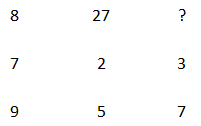Question 4

# Select the missing number from the given options.Solution

In the given table,

The pattern is in all the column is same,

First-term of first column $$8=2^3$$  and third term =(second term)$$7+2=9$$

First-term of second column $$27=3^3$$ and third term =(second term)$$2 +3=5$$

So, first term of the third column will $$7-3=4$$ Hence, the first term will be $$4^3=64$$

Hence, option A is the correct answer.

• Free SSC Study Material - 18000 Questions
• 230+ SSC previous papers with solutions PDF